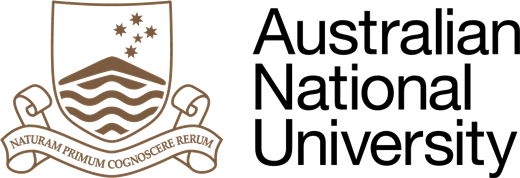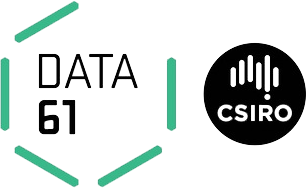## The BusPlus Project

### Connecting people

Canberra was founded in 1913 by American architect Walter Burley Griffin, it is a vast, planned city built as a collection of towns separated by green belts.

• Canberra: 381,488 hab.
814.2 km² (428.6/km²)
• Montréal (City): 1,649,519 hab.
431.50 km² (4,517.6/km²)
• Montréal (Metro): 4,127,100 hab.
4,258.31 km² (898.1/km²)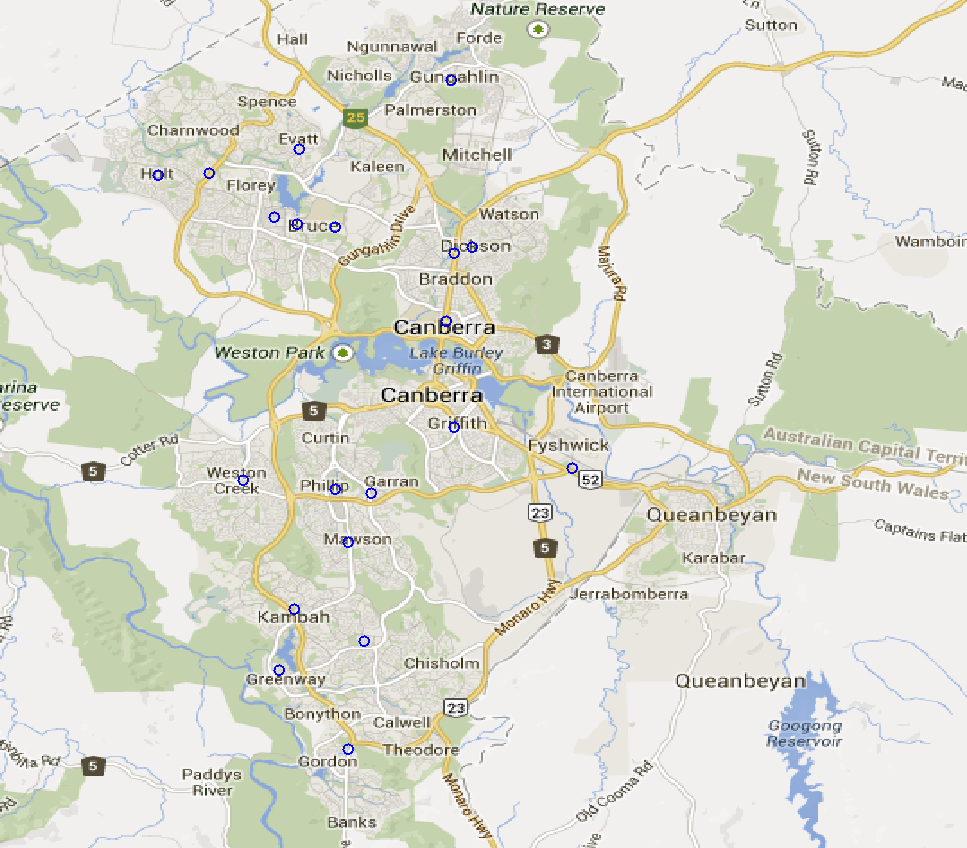## Why BusPlus?

### Action

• Demand is too spread out
• Most buses run almost empty
• Very high cost: almost 100 bus lines
• Service is not regular, esp. off-peak

### BusPlus

• Booking system, trips start at a bus stop
• Low waiting time (15min preparation time)
• High frequency buses (aiming for every 15min)
• Shuttles for "first" and "last mile"

## Modelling BusPlus

### The data

• One week of data (Monday to Saturday)
• 2,800 bus stops
• 21,000 trips for 60,000 passengers
• Two sets of hubs: $H_{10}$ and $H_{20}$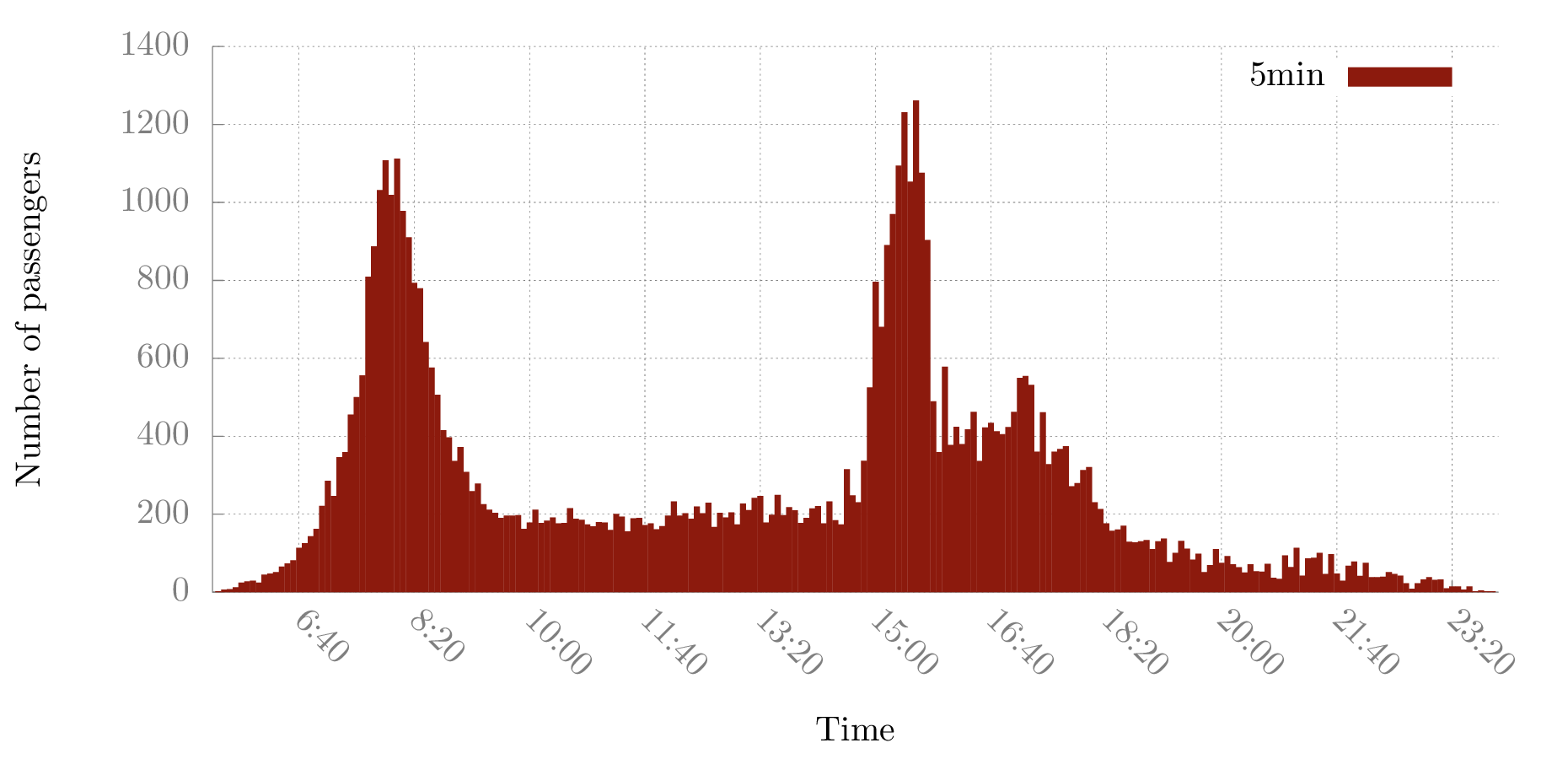## Modelling BusPlus

### Hub Location and BusPlus

The Hub-Arc Location Problem  (HALP) is a variant of the well-known Hub Location Problem where hubs do not form a complete graph.## Modelling BusPlus

### Hub Location and BusPlus

The Hub-Arc Location Problem  (HALP) is a variant of the well-known Hub Location Problem where hubs do not form a complete graph.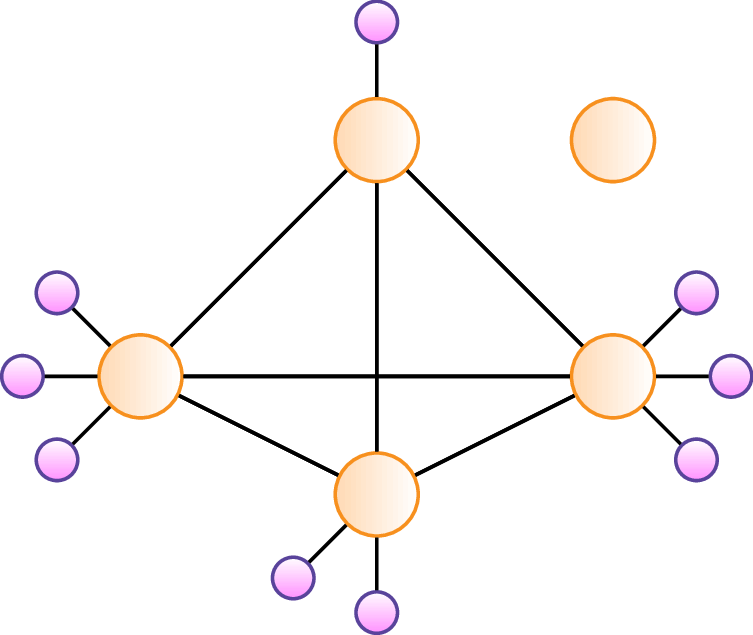Campbell, J.F., Ernst, A.T. and Krishnamoorthy, M., 2005.

Hub arc location problems: part I & II.

Management Science, 51(10), pp.1540-1571.

## Modelling BusPlus

### Hub Location and BusPlus

The Hub-Arc Location Problem  (HALP) is a variant of the well-known Hub Location Problem where hubs do not form a complete graph.

The HALP is used when designing networks where opening links between hubs has a cost but these links provide economies of scale (e.g., transhipping networks).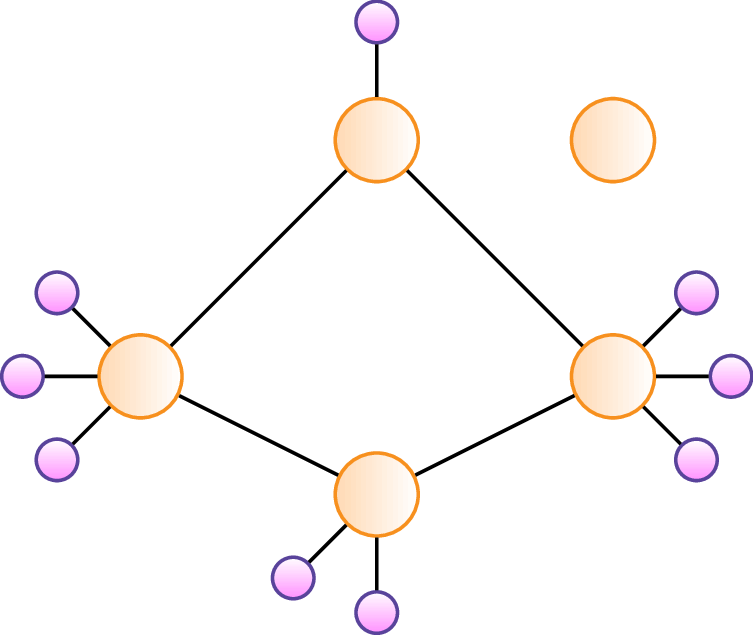Campbell, J.F., Ernst, A.T. and Krishnamoorthy, M., 2005.

Hub arc location problems: part I & II.

Management Science, 51(10), pp.1540-1571.

## Modelling BusPlus

### Variables and costs

• Costs is a mix of expenses and time, conversion factor $\alpha$
• Taxis $x$ available everywhere to route trips, combination of time and cost: $$\tau = (1 - \alpha) d_{ij} \cdot T + \alpha \cdot t_{ij}$$
• Bus legs (hub arcs) $z$ with a fixed installation cost: $$\beta = (1 - \alpha) d_{hl} \cdot B$$
• Bus trips $y$ between hubs, only cost $$\gamma = \alpha (t_{ij} + S)$$## Modelling BusPlus

### Hub-and-Shuttle Public Transportation System (HSPTS)

\begin{align} \min && \sum_{r \in T} \sum_{i,j \in N} \tau_{ij}^r x_{ij}^r + \sum_{r \in T}\sum_{h, l \in H} \gamma_{hl}^r y^r_{hl} & + \sum_{h,l \in H} \beta_{hl} z_{hl} \\ s.t. && \sum_{l \in H} z_{hl} & = \sum_{l\in H} z_{lh} & \forall h \in H \label{eq:hub} \\ & & y^r_{hl} & \leq z_{hl} & \forall r\in T, \forall h,l \in H \label{eq:hard} \\ & & \sum_{j \in N} \left( x^r_{ij} - x^r_{ji} \right) + \sum_{\substack{h \in H \\ \text{if } i \in H}} \left(y^r_{ih} - y^r_{hi} \right) & = 1, 0, -1 & \forall r \in T, \forall i \in N \\ & & x_{ij}^r, y_{hl}^r \geq 0; z_{hl} \in \B \end{align}

## Trimming the Model

### Warming up, because not all taxis are born equal...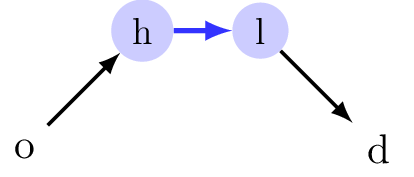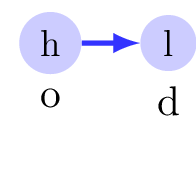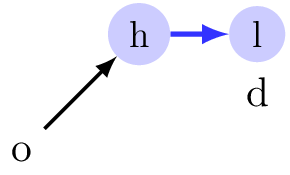### T-Filtering

Some trips will never use the bus network, whatever the configuration!

About 30% of trips can just be omitted in the model when using 10 hubs; 20% with 20 hubs.

### L-Filtering

We have an upper bound on the cost $\tau_{od}$, let us remove any "best" scenario above that:

if $\tau_{oh} + \gamma_{hl} + \tau_{ld} > \tau_{od}$, then remove $x_{oh}$ and $x_{ld}$.

55% to 58% reduction in the number of $x$ variables.

$H_{10}$ has 130,000 $x$ variables, $H_{20}$ has 300,000.

## Benders Decomposition

### What is it?

• Take a MIP
• Project out continuous variables
• You obtain two problems: a master and a sub-problem

### BusPlus

The HALP can actually be seen as a two-level decision process.

1. 1. Which arcs to open
2. 2. How to route the flow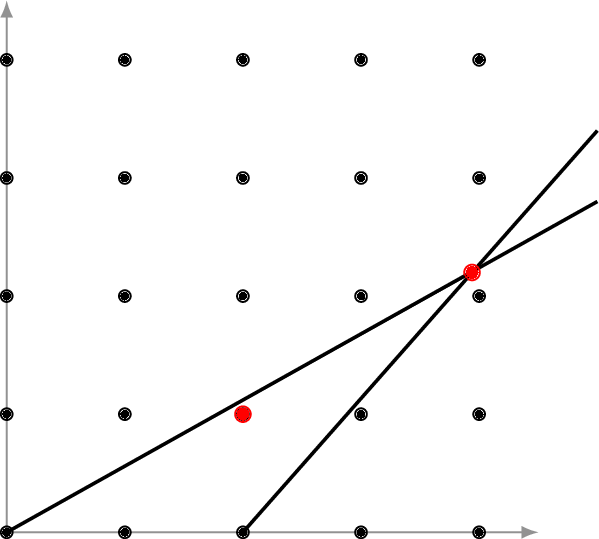## Benders Decomposition

### How does it work?

• The master is a relaxed problem, it fixes the integer variables
• Solve the dual LP with the candidate solution to generate cuts
• Instead of having to explore all solutions, only add "relevant" constraints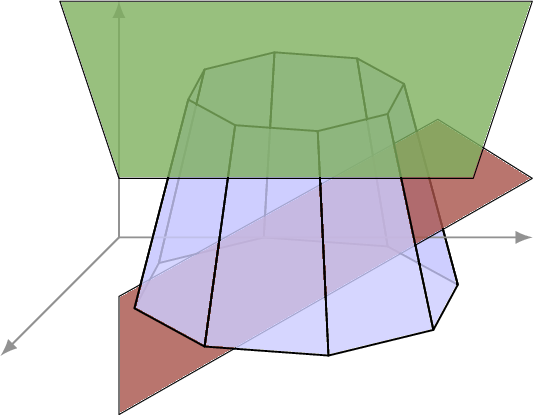## Benders Decomposition

### Master

\begin{align} \min && \sum_{h,l \in H} \beta_{hl} z_{hl} + q \nonumber \\ s.t. && \sum_{l \in H} z_{hl} = \sum_{l \in H} z_{lh} &\ \forall h \in H \\ && \text{A set of cuts}\\ && z \in \B, q \in \R \end{align}

### Sub-Problem

\begin{align} \min && \sum_{r \in T} \left( \sum_{i, j \in N}% \tau_{ij}^r x_{ij}^r +% \sum_{h, l \in H} \gamma_{hl}^r y_{hl}^r \right) \\ s.t. && y^r_{hl} \leq \bar{z}_{hl} \quad \forall r \in T, h,l \in H\\ && \sum_{j \in N} \left( x^r_{ij} - x^r_{ji} \right) + \sum_{\substack{h \in H \\ \text{if } i \in H}} \left(y^r_{ih} - y^r_{hi} \right) = 1, 0, -1 \\ && \forall r \in T, \forall i \in N \\ && 0 \leq x_{ij}^r, y_{hl}^r \leq 1 \end{align}

## Benders Decomposition

### Master

\begin{align} \min\quad \sum_{h,l \in H} \beta_{hl} z_{hl} + q \nonumber \\ s.t.\quad \sum_{l \in H} z_{hl} = \sum_{l \in H} z_{lh} &\quad \forall h \in H \\ u_o - u_d - \sum_{h, l \in H} z_{hl} v_{hl} \geq q &\quad\! \forall (u, v) \in \mathcal{O} \\ z \in \B, q \in \R \end{align}

### (Independent) Dual Sub-Problem

\begin{align} \max && u_o - u_d - \sum_{h, l \in H} & \bar{z}_{hl} v_{hl} \\ s.t. && u_i - u_i & \leq \tau_{ij} & \forall i,j \in N \\ && u_h - u_l - v_{hl} & \leq \gamma_{hl} & \forall h,l \in H \\ && u, v \geq 0 \end{align}

## Improving Benders

### Pareto optimal cuts 

One issue with min-cost flow, as with many "network" problems, is its high degeneracy: multiple equivalent optimal solutions.

So we design a Pareto optimal sub-problem.

• Modify the objective function using a core point
• Add a constraint to restrict the solution space with the solution of the primal problem
\begin{align} \max && u_o - u_d - \sum_{h,l \in H} & z^0_{hl} v_{hl} \\ s.t. && u_i- u_j & \leq \tau_{ij} \quad \forall i,j \in D^r \\ && u_h - u_l - v_{hl} & \leq \gamma_{hl} \quad \forall h, l \in H \\ && u_o - u_d - \sum_{h,l \in H} \bar{z}_{hl} v_{hl} &= spp(o, d, \bar{Z}) \\% && u \geq 0, v \geq 0 \end{align}

 Magnanti, T.L. and Wong, R.T., 1981.

Accelerating Benders decomposition: Algorithmic enhancement and model selection criteria.

Operations research, 29(3), pp.464-484.

## Improving Benders

### Pareto optimal cuts and independent sub-problems## Improving Benders

### Cut bundling

With fixed $z$, the sub-problem becomes a collection of independent min-cost flows.

We need to decide how we are going to "bundle" the cuts.

• All in one
• By origin
• By leg used
• By closest hub
• One per trip## Improving Benders

### Core point update

Papadakos  showed that the choice of a core point can dramatically influence the performance of Pareto approach. Even better when updating it. $$z^{0 (n + 1)}_{hl} = \frac{z^{0 (n)}_{hl} + \bar{z}^{(n)}_{hl}}{2}$$Integrated airline scheduling.

Computers & Operations Research, 36(1), pp.176-195.

## Results

### Benders vs. MIP (small)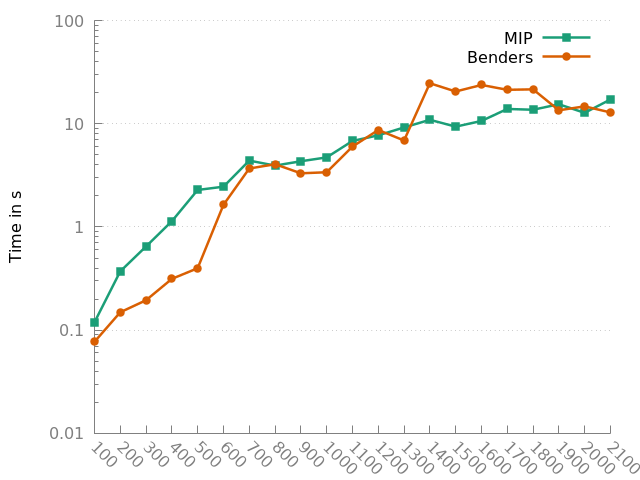## Results

### Benders vs. MIP (large)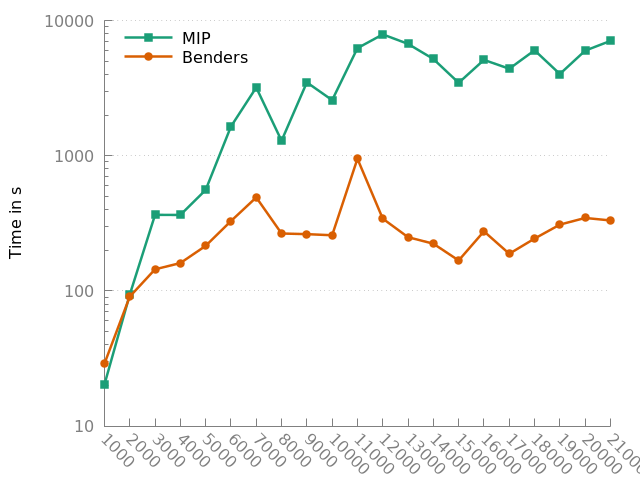## Results

### Sensitivity analysis